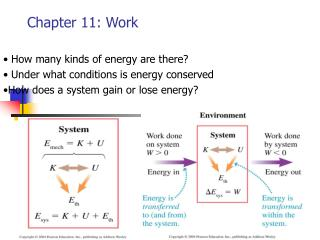DownloadDownload PresentationChapter 11: Work

# Chapter 11: Work

Download Presentation## Chapter 11: Work

- - - - - - - - - - - - - - - - - - - - - - - - - - - E N D - - - - - - - - - - - - - - - - - - - - - - - - - - -
##### Presentation Transcript

1. Chapter 11: Work • How many kinds of energy are there? • Under what conditions is energy conserved •How does a system gain or lose energy?

2. Stop to think 11.1 P304Stop to think 11.2 P306Stop to think 11.3 P310Stop to think 11.4 P312Stop to think 11.7 P327 • Example 11.1 P307 • Example 11.6 P311 • Example 11.8 P313

3. Work and kinetic energy Force F does work as the particle moves from Si to Sf

4. The work-kinetic energy teorem • We integrate both sides Question: 1 Kg ball free fall from 5m high place to the ground, how much work is Done by the gravitational force?

5. Calculating work • Constant Force • Θ is the angle between force and displacement.

6. Calculating the work of a constant force.

7. The work done by a force depends on the angle θ between the force and displacement, not on the direction the particle is moving. The work done on all four particles in the figure is the same, despite the face that they are moving in four different direction.

8. The dot product of two vectors • Dot product: • It is scalar • We also can do dot product using components

9. The work done by a variable force • From the definition of work • (1) Do integral • (2) Find the area under the force-vs –position graph • Ex. The spring force is a variable force F = -kx ( we chose equilibrium position x(e) as origin )

10. Work and potential energy • Conservative force: A force for which the work done on a particle as it moves from an initial to final position is independent of the path For example • Gravitational force is conservative force, If it does positive work, always make potential energy decrease. • Gravitation force and spring force are conservative force. Independent of path

11. Nonconservative force • A force for which the work is not independent of the path is called a nonconservative force. For example, friction. • If there are only conservative forces because Wc = ΔK =-ΔU→ ΔK + ΔU =0 ( total mechanical energy is conserved) • If there is nonconservative force ΔK + ΔU = Wnc or

12. Problem 11.49 • Use work and energy to solve the problem. • (a) the table is frictionless, W(fric)=0 • Ei = m2 gh + m1 gy1 • Ef =1/2 (m1+m2)V2 +m1 g y1 • From Ei = Ef, you can find V • (b) if there is a friction force between m1 and table. f(k) = μ(m1g) Ei – f(k)h = Ef m2 gh -μ(m1g)h = ½(m1+m2)V2 m1 m2 y1

13. P11.56 The spring shown in Fig. is compressed 50cm and used to launch a 100 Kg students. The track is frictionless until it stars up the incline. The student’s coefficient of kinetic friction on the 30o incline is 0.15 • The system is compressed spring, student and the ground. • Just before and after student is losing the contact with the spring, the energy conservation equation is: • How far up the incline does the student go? V2=0 h1 h2

14. How far up the incline does the student go? • The total energy at top of 10 m should equal the total energy at bottom. After that student goes to incline with friction. We can apply the energy and work equation. With h2 = s sin(30o) and friction F(k) = μ mg cos(30o) Get h1 h2

15. Finding force from potential energy • The work done by conservative force is

16. Power • Power: rate of transfer of energy is defined as: unit of power is Watt. When work as the source of energy trasfer